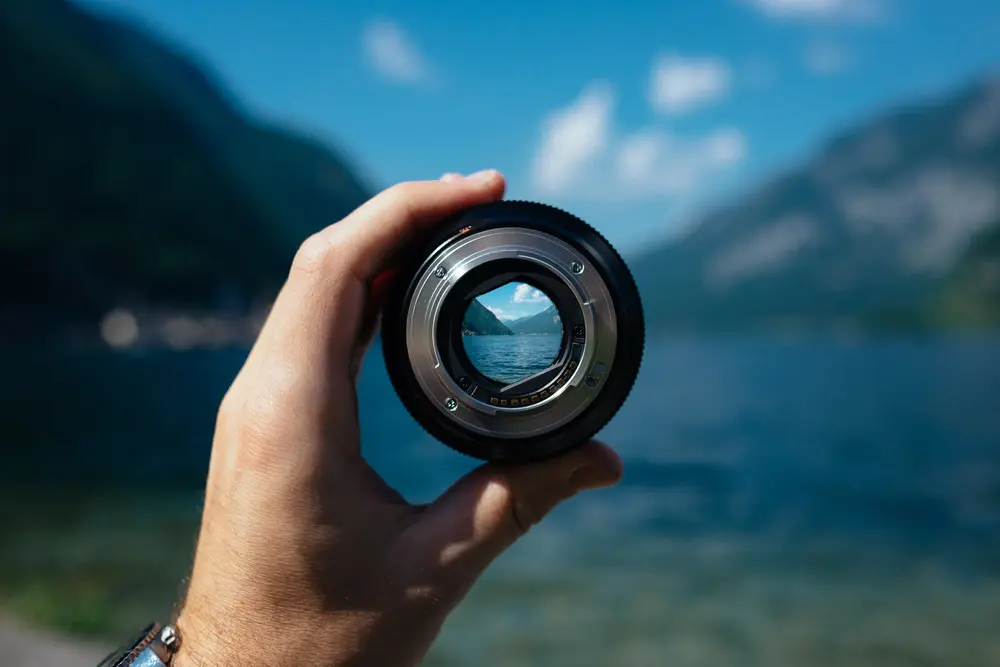# Lenses

• A lens its self is a very simple device, it is just a part of glass (or another transparent material) which unevenly shaped. When light passes from air to a denser material it slows down. In a concave lens the light has to travel further through the middle then through the sides. This has the affect of pushing the waves back in the middle and forward around the edge therefore effectively adding curvature to the wave. A similar thing happens when passing through a concave lens but obviously vice-versa, taking away curvature of the wave.
• The curvature that the lens adds or takes away is the Power of the lens, measured in dioptres. P=1/f, P is the power of the lens and f is the focal length. The focal length of a lens is the distance from a lens to its focal point, which is where the image of a distant object is formed. The shorter the focal length the more powerful the lens.
• The Cartesian sign convention, shows how much curvature a lens adds to an image, and the formulae can be rearranged to give where the image will be focused.
• 1/v=1/u + 1/f
• Where v is the distance from the lens to its focal point, u is the distance from the object to the lens and 1/f is the power of the lens. This follows from the above, the power shows how much curvature is added to the wave. As a wave moves further away from an object the curvature of it decrease, imagine a circle as the radius gets larger the curvature in one particular area will decrease. Therefore the curvature of the wave is the inverse of the distance away from the object, and the curvature of the image is again the inverse of the distance it is away from the lens. You may need to read that again if you don’t fully grasp this concept.
• The magnification of a lens is something that even if you don’t know, you should really understand immediately.
• m=v/u (when in focus)
• Where m is the magnification, v is the distance from the lens to the image and u is the distance from the lens to the object. The further away the image is from the lens the higher the magnification, and the closer the image is to the lens the higher the magnification. This is common sense, and you will quickly understand this if you use a lens for a few minutes.
Imaging

Imaging

Imaging

Imaging

Imaging